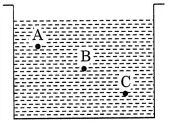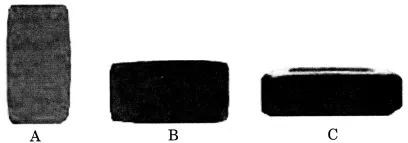# CBSEtips.in

## Wednesday 24 February 2021

### CBSE Class 8 Science - MCQ and Online Tests - Unit 11 - Force and Pressure

#### CBSE Class 8 Science – MCQ and Online Tests –  Unit 11 – Force and Pressure

Every year CBSE students attend Annual Assessment exams for 6,7,8,9,11th standards. These exams are very competitive to all the students. So our website provides online tests for all the 6,7,8,9,11th standards’ subjects. These tests are also very effective and useful for those who preparing for any competitive exams like Olympiad etc. It can boost their preparation level and confidence level by attempting these chapter wise online tests.

These online tests are based on latest CBSE syllabus. While attempting these, our students can identify their weak lessons and continuously practice those lessons for attaining high marks. It also helps to revise the NCERT textbooks thoroughly

#### CBSE Class 8 Science – MCQ and Online Tests – Unit 11 – Force and Pressure

Choose the correct option in the following questions:

Question 1.
During dry weather, while combing hair, sometimes we experience hair flying apart. The force respon毗ible for this is
(a) force of gravity
(b) force of friction
(c) electrostatic force
(d) magnetic force

Question 2.
A spring balance is used for measuring
(a) mass
(b) weight
(c) pressure
(d) speed

Question 3.
A container is filled with water as shown in the given figure. Which of the following statements is correct about pressure of water?(a) Pressure at A > Pressure at B > Pressure at C
(b) Pressure at A = Pressure at B = Pressure at C
(c) Pressure at A < Pressure at B > Pressure at C
(d) Pressure at A < Pressure at B < Pressure at C

Answer: (d) Pressure at A < Pressure at B < Pressure at C

Question 4.
An example of a non- contact force is
(a) Force exerted by us to lift a bucket
(b) Push a stationary car
(c) Force exerted by magnet
(d) Hit a cricket ball for a 6 run

Answer: (c) Force exerted by magnet

Question 5.
A ___________ exerted by an object on another is a force.
(a) Push or pull
(b) Contact or non-contact force
(c) Pressure
(d) Magnitude

Question 6.
The force exerted by the earth to pull the object towards itself is called
(a) electrostatic force
(b) gravitational force
(c) muscular force
(d) contact force

Question 7.
Muscular force is also called ___________ force.
(a) non-contact
(b) contact
(c) gravitational
(d) magnetic

Question 8.
The force exerted by a charged body on another char
(a) gravitational force
(b) electrostatic force
(c) non-contact force
(d) contact force

Question 9.
Force changes the
(a) motion of body
(b) speed of body
(c) shape of body
(d) all of these

Question 10.
The force exerted by our muscle is called
(a) electrostatic force
(b) muscular force
(c) gravitational force
(d) non-contact force

Question 11.
1 kilogram weight is equal to
(a) 98 N
(b) 9.8 N
(c) 0.98 N
(d) 0.098 N

Question 12.
Two boys A and B are applying force on a block. If the block moves towards the boy A, which one of the following statements is correct?
(a) Magnitude of force applied by A is greater than that of B.
(b) Magnitude of force applied by A is smaller than that of B.
(c) Net force on the block is towards B.
(d) Magnitude of force applied by A is equal to that of B.

Answer: (b) Magnitude of force applied by A is smaller than that of B.

Question 13.
The pressure which is exerted by air around us is known as
(a) force
(b) atmospheric pressure
(c) muscular force
(d) friction

Question 14.
Force acting on per unit area is called
(a) non-contact forces
(b) contact forces
(c) force
(d) pressure

Question 15.
Leaves fall down on the ground due to
(a) electrostatic force
(b) magnetic force
(c) gravitational force
(d) muscular force

Question 16.
State of motion is described by
(a) Position of rest
(b) Position of motion
(c) Both by the state of rest or motion
(d) None of these

Answer: (c) Both by the state of rest or motion

Question 17.
When the hammer strikes the gong of an electric bell, which of the following force is responsible for the movement of hammer?
(a) Gravitational force alone
(b) Magnetic force alone
(c) Electrostatic force alone
(d) Frictional force alone

Question 18.
Two objects repel each other. This repulsion could be due to
(a) frictional force only
(b) electrostatic force only
(c) magnetic force only
(d) either a magnetic or an electrostatic force

Answer: (d) either a magnetic or an electrostatic force

Question 19.
Pressure =
(a) Area / force on which it acts
(b) force / area on which it acts
(c) Volume / force on which it acts
(d) Force / volume on which it acts

Answer: (b) force / area on which it acts

Question 20.
Gravity is
(a) Repulsive
(b) Attraction + Repulsive force
(c) Attractive force
(d) Not a force

Question 21.
Which one of the following forces is a contact force?
(a) Force of gravity
(b) Magnetic force
(c) Force of friction
(d) Electrostatic force

Question 22.
A brick is kept in three different ways on a table as shown in given figure. The pressure exerted by the brick on the table will be(a) maximum in position A
(b) maximum in position C
(c) maximum in position B
(d) equal in all cases

Answer: (a) maximum in position A

Question 23.
A push or pull on an object is called
(a) Pressure
(b) Push-pull
(c) Force
(d) All of the above

Question 24.
If two forces act in the opposite directions on an object, the net force acting on it is the
(a) Sum of the two forces
(b) difference between the two forces
(c) Multiplication of the two forces
(d) Division of the two forces

Answer: (b) difference between the two forces

Question 25.
Which of the following is proper example(s) to explain that force on an object may change its shape
(a) A ball of dough rolled into chapati
(b) Pressing a rubber ball kept on table
(c) Making model using clay
(d) All of the above

Answer: (d) All of the above

Question 26.
A ball rolling on the ground slows down and finally stops. This is because of
(a) Force
(b) Less force applied
(c) Friction
(d) None of the above

Question 27.
Force of friction always acts on moving objects and its direction shall be ____
(a) On any direction
(b) Along the direction of motion
(c) Perpendicular to the direction of motion
(d) Opposite to the direction of motion

Answer: (c) Perpendicular to the direction of motion

Question 28.
If In a tug-o-war, when two teams are pulling a rope, and the rope does not move towards any team, it implies that
(a) Equal force is being applied in the same direction
(b) Equal Force is being applied in opposite direction
(c) No force is applied in any direction
(d) Cannot be explained

Answer: (b) Equal Force is being applied in opposite direction

Question 29.
When two forces act in opposite directions, then net force acting is the
(a) sum of two forces
(b) difference between two forces
(c) both of these
(d) none of these

Answer: (b) difference between two forces

Question 30.
The strength of force is expressed by its
(a) weight
(b) mass
(c) magnitude
(d) longitudinal force